# Evaluating a double integral

• docnet

#### docnet

Homework Statement
Evaluate ∫∫ -4 / (1+ x^2 + y^2)^4 dx dy
Relevant Equations
∫∫ -4 / (1+ x^2 + y^2)^4 dx dy
Dear all,

Last semester on the final exam, our professor gave us an integral that seems difficult to solve.

The integral came at the end of a lengthy problem, where we were asked to find the net Gauss curvature of Enneper's surface.

The integral that emerged is the following.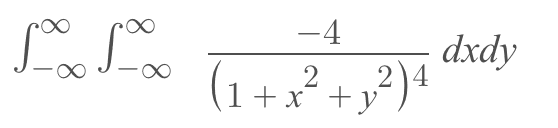We tried substituting x = sinθ, y = cosΘ and ended up with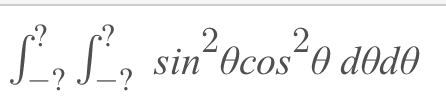which does not help.

We tried evaluating the following single integral to see if it simplifies.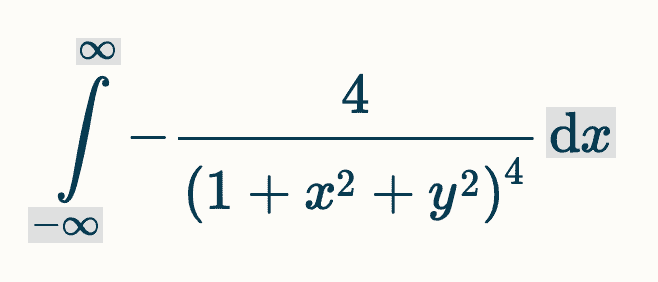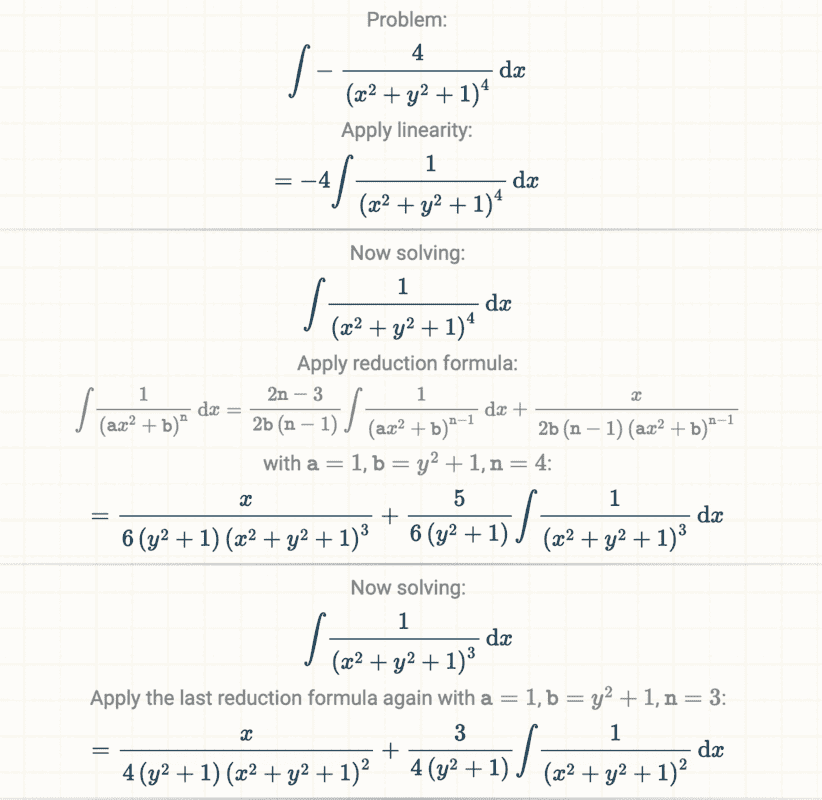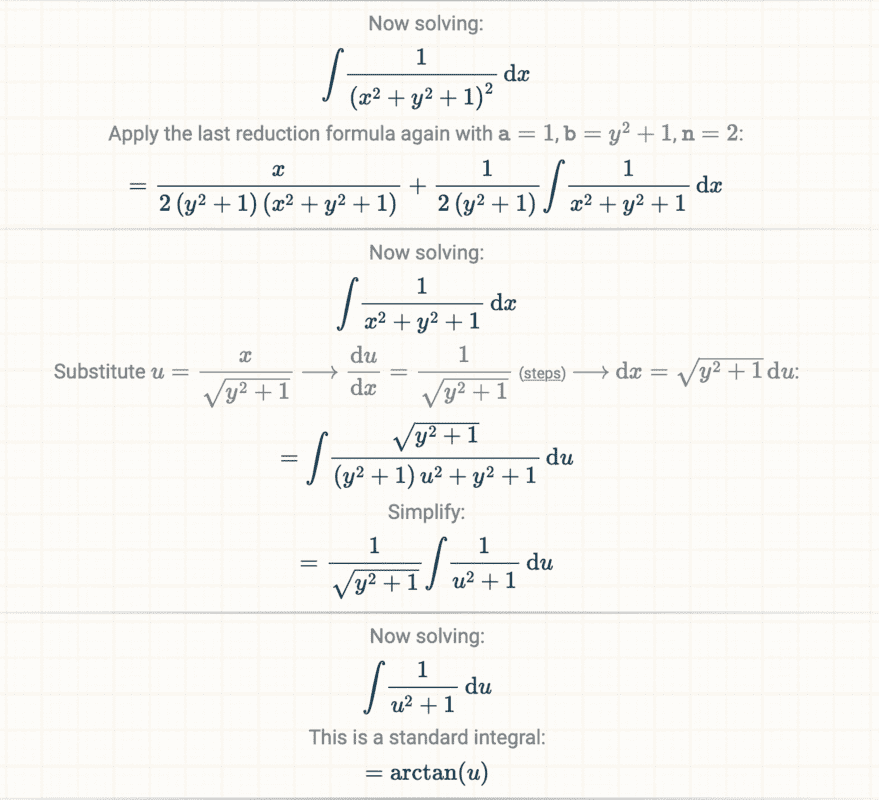...

And the calculations continue for another 3 pages and become more complicated.

The evaluated integral is the following.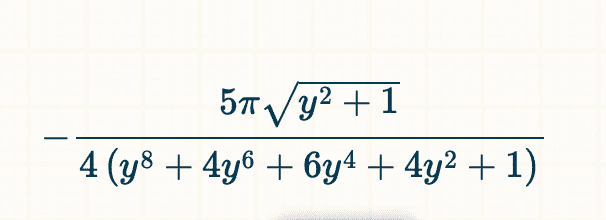We could integrate this expression with respect to y and evaluate the result from -∞ to ∞ .

The answer for the net curvature is - 4π in differential geometry textbooks.

Is there a key integration technique we are missing?

This integral seems difficult to solve without using technology.

Thank you.

Homework Statement:: Evaluate ∫∫ -4 / (1+ x^2 + y^2)^4 dx dy
Relevant Equations:: ∫∫ -4 / (1+ x^2 + y^2)^4 dx dy

Change to polar coordinates.

•docnet
We tried substituting x = sinθ, y = cosΘ
Change to polar coordinates.
I agree with George. You almost had it, @docnet.

•docnet
Dear @Mark44 and @George Jones,

We substituted x = r sinΘ, y = r cosΘ and used the chain rule to determine dx and dy.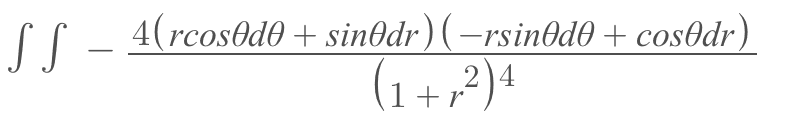The new boundaries of integration in polar coordinates are determined by the sets {0 ≤ r ≤ ∞} and {0 ≤ Θ ≤ 2π}.

We then expanded the numerator to obtain the following integrals.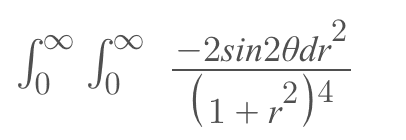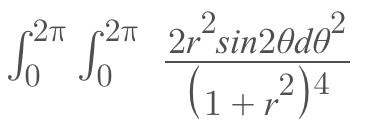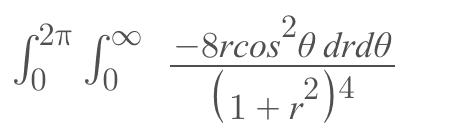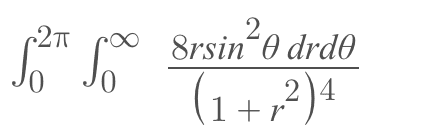Evaluating the first integral

We try substituting r = tan2u to simplify the process, and treat sin2Θ as a constant. The new boundaries of integration are determined by the set {0 ≤ u ≤ .5π} and we have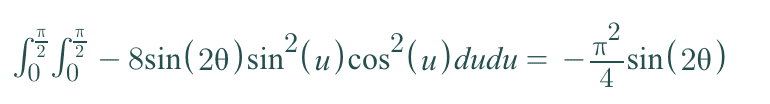to be continued.

When you convert from rectangular to polar, dxdy becomes ##rdrd\theta##, so the numerator will become ##-4rdrd\theta##.

•docnet
@docnet , what is the area bounded by the following four curves:
a line from the origin at angle ##\theta##;
a line from the origin at angle ##\theta + d\theta##.

Draw a diagram to see.

When you convert from rectangular to polar, dxdy becomes ##rdrd\theta##, so the numerator will become ##-4rdrd\theta##.

@Mark44
Thank you for pointing out my error. This would have been a time sink.

So we have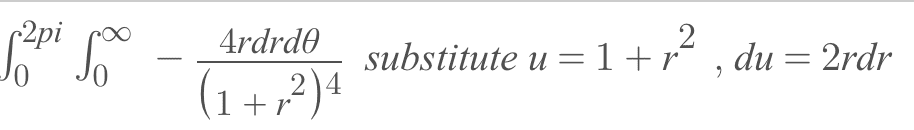and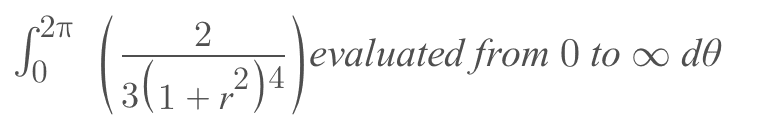and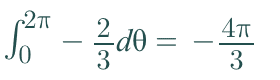Which is our net Gaussian curvature of Enneper's Surface.

Last edited:
@docnet , what is the area bounded by the following four curves:
a line from the origin at angle ##\theta##;
a line from the origin at angle ##\theta + d\theta##.

Draw a diagram to see.

@ George Jones

The area bounded by the four curves is represented in the following diagram, the area around the purple dot. The distance between the two red circles is dr and the angle created by the two lines is dΘ.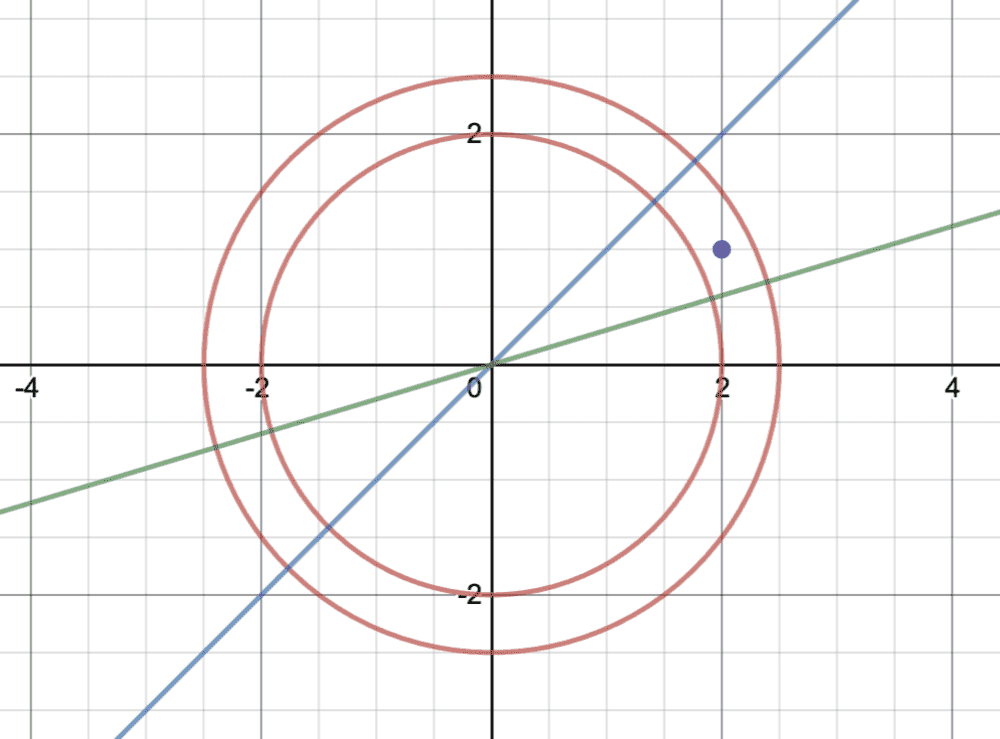The area bounded by the four curves is represented in the following diagram, the area around the purple dot.

View attachment 275654
Yes. What does this area equal?

Yes. What does this area equal?

@George Jones,

Integrating 1 to determine the area, we have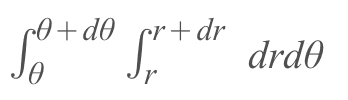=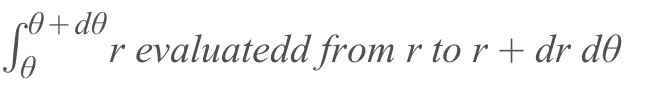=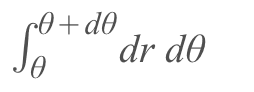=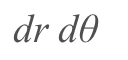The textbook gives the net curvature of Enneper's surface as - 4π but frankly I don't know if that is equal to the net Gaussian curvature. I assume they are different.

Let's do this a little more intuitively. Since ##dr## and ##d\theta## are "infinitesimally small", the bounded area is a "rectangle". What are the lengths of its sides?

Let's do this a little more intuitively. Since ##dr## and ##d\theta## are "infinitesimally small", the bounded area is a "rectangle". What are the lengths of its sides?

Since a length of an arc of a circle is the radius times the angle, we have a rectangle of side dr and side rdΘ. length rdΘ corresponds to the side perpendicular to the radial direction.

The corresponding area is r drdΘ, that is congruent to our infinitesimally small area element dydx in rectangular coordinates.

•docnet
@Delta2.

The description of the parallelogram in the wikipedia link feels reminiscent of the geometric representation of the determinant of a 2 x 2 matrix.

In differential geometry, we learned the area factor corresponding to the metric is determined by taking the square root of the determinant of the metric.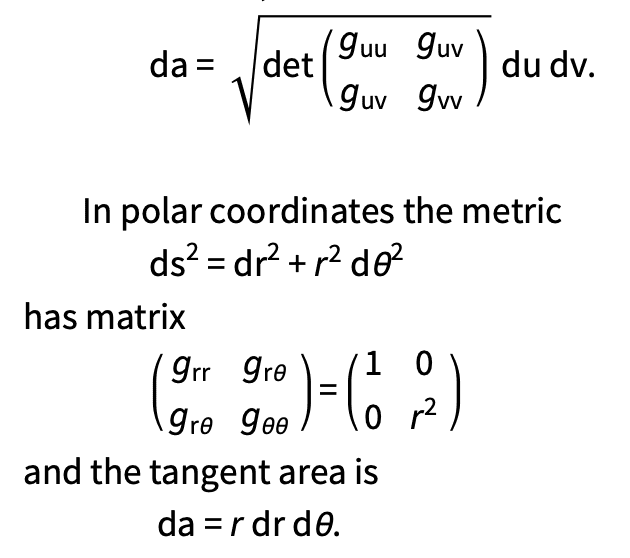I assume this is equivalent to the Jacobian method you mentioned?

Ok, I frankly don't know the method you are saying, it uses the square root of the determinant of a different matrix (not the Jacobian matrix) but it gives the same result, at least for two variables.

•docnet
oh ok. I think if we had time, we could show the Jacobian matrix and the matrix of the metric are at least related somehow.

The Jacobian matrix is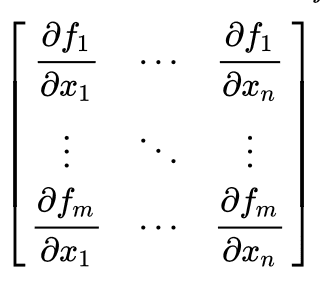The area element corresponding to the metric is determined by taking the partial derivatives of a map

x = r sinΘ
y = r cosΘ

dx = rcosΘdΘ + sinΘdr
dy = -rsinΘdΘ + cosΘdr

and computing dx2 + dy2 = dr2 +r22

The diagonal elements of the matrix corresponding to our metric are 1 and r2, and there are no off-diagonal elements since there are no cross terms in our metric.

Its determinant is simply r2 whose square root is r.

So r is the factor of integration whenever we switch from euclidian coordinates to polar coordinates. I assume dr and just follow.

We haven't learned about using the Jacobian to do change of variables in an integral. We only learned to use the Jacobian to give sufficient conditions in certain optimization problems in class.

if ##J## is the jacobian matrix as you have it in post #17 then it is $$df_1df_2...df_n=|det(J)|dx_1dx_2...dx_n$$ when we change the variables from ##f_1,f_2,...,f_n## to ##x_1,x_2,...,x_n##.

•docnet
Dear @Delta2,

so we have

x = r sinΘ
y = r cosΘ

whose Jacobian is

rcosΘ sinΘ
-rsinΘ cosΘ

and the determinant is

rcos2Θ + rsin2Θ = r

which gives our change of variables from xy space to polar coordinates.

dxdy = > rdrdΘ

•Delta2